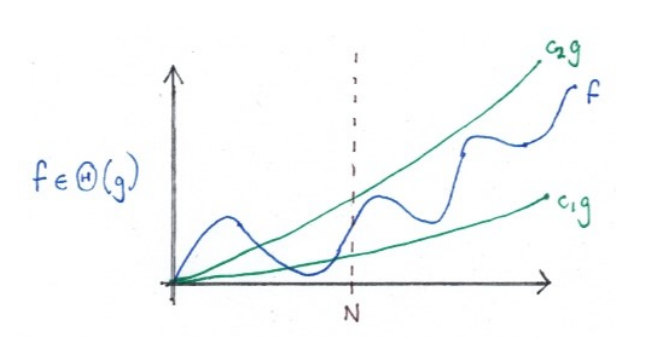our main character: o, ω, O, Ω and Θ

## DEFINITION

Asymptotic Notation
Asymptotic notations are the mathematical notations used to describe the running time of an algorithm when the input tends towards a particular value or a limiting value.

## Why we use asymptotic notation instead of detailed run-time function?

1. :fire: TRIGGER:
• The function describe algorithm is too complicate to see how an algorithm performs.
• We’re only interested in the broad headlines of how some function behaves。
2. :star: MOTIVATION:
• We find it is possible to classify these ‘complicate’ functions via ‘simple’ ones.
3. :boom: ATTAMPTS:
• Asymptotic Notation :heavy_check_mark:
4. :sparkles: CONSEQUENCE:
• Reduce clutter and simplify calculation.
• Way of making precise, quantitative statements about efficiency properties of algorithms themselves.

## How to use asymptotic notation?

There are 5 different asymptotic notation: o, ω, O, Ω and Θ. And they share some PROPERTIES.

1. $x(g) = {f : N → R≥0 | {definition} {of} x}$ , where x is one of these five.
2. So, f is x(g) technically means f ∈ x(g).

Then let’s see how to use each of them

• Example case
• f = o(g)
• How to say
• f is asymptotically smaller than / grows slower than g
• In the limit, f is vanishingly small relative to g
• Definition
• ∀c > 0. ∃N. ∀n ≥ N. f (n) < cg(n)
• Property
• A robust statement about f and g. E.g. unaffected by scaling: f = o(g) ⇔ 3f = o(0.2g)
• Memory tips
• Little o
• g 在增长级数上碾压 f
• Example case
• f = ω(g)
• How to say
• g is asymptotically larger than / grows faster than f
• Definition
• ∀C > 0. ∃N. ∀n ≥ N. f (n) > Cg(n)
• Property
• A robust statement about f , g. Same as Little o.
• f = o(g) ⇔ g = ω(f )
• Memory tips
• Think ‘C = 1/c’
• Compare: x < y if and only if y > x.
• g 增长级数上永远小于 f
• Example case
• f = O(g)
• How to say
• f is O(g) ‘f grows no faster than g’
• call g an asymptotic upper bound for f
• Definition
• ∃c > 0. ∃N. ∀n ≥ N. f (n) ≤ cg(n)
• Property
• f = o(g) implies f = O(g)
• Notice
• Different with Little o:
• For o we require that any multiple of g eventually overtakes f .
• For O it’s enough that some multiple of g does.
• ∀c compare with ∃C
• If f = O(n^2) is a tight upper bound, f = O(n^3) is true but not a - tight upper bound.
• Memory tips
• Big O
• Loosely, can think of o as like <, O as like ≤.
• g 在增长级数上和 f 类似，但会存在 g 的增长率大于 f 的情况
• Example case
• f = Ω(g)
• How to say
• f is Ω(g) ‘f grows no slower than g’
• g is an asymptotic lower bound for f
• Definition
• ∃C > 0. ∃N. ∀n ≥ N. f (n) ≥ Cg(n)
• Property
• f = O(g) ⇔ g = Ω(f )
• Memory tips
• Ω(g) ≤ Θ(g) ≤ O(g)
• g 在增长级数上和 f 类似，但会存在 g 的增长率小于 f 的情况
• Example case
• f = Θ(g)
• How to say
• f and g have ‘essentially the same growth rate’
• g is an asymptotically tight bound for f
• Definition
• ∃c1, c2 > 0. ∃N. ∀n ≥ N. c1g(n) ≤ f (n) ≤ c2g(n)
• Property
• f = Θ(g) ⇔ g = Θ(f )
• f is Θ(g) ⇔ f is O(g) and f is Ω(g)
• Memory tips## Other

• for some runtime function T(n):
• T(n) = O(g) says runtime is not essentially worse than g(n),
• T(n) = Ω(g) says runtime is not essentially better than g(n).

If you have better memory tips, tell my in the comment. It will be great.